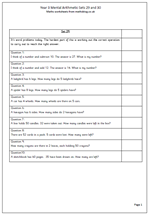# Year 3 mental arithmetic: sets 29 and 30This week the questions are all in the form of word problems. Generally children find these harder than just ‘sums’ because they are not told what operation needs to be carried out to reach the correct answer. Indeed, sometimes it can be tricky. Looking at the question,

‘I think of a number and subtract 10. The answer is 27. What is my number?’

Immediately the word subtraction will come to mind as it is used in the question. But, in fact, an addition has to be done to find the correct answer.

Several questions also require a good knowledge of the 2x, 5x and 10x tables.

If you are reading these out for children to answer be sure to read each question slowly and at least twice. The first time the question is heard the child will probably be thinking about what needs to be done. The second time reinforces the numbers involved.

Year 3 mental arithmetic (sets 29 and 30)